##### Signals and Systems For Dummies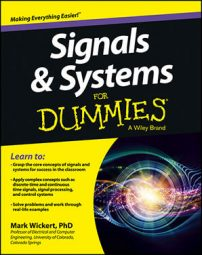A signal is classified as deterministic if it’s a completely specified function of time. A good example of a deterministic signal is a signal composed of a single sinusoid, such as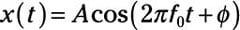with the signal parameters being: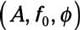A is the amplitude, f0 is the frequency (oscillation rate) in cycles per second (or hertz), and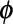is the phase in radians. Depending on your background, you may be more familiar with radian frequency,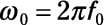which has units of radians/sample. In any case, x(t) is deterministic because the signal parameters are constants.

Hertz (Hz) represents the cycles per second unit of measurement in honor of Heinrich Hertz, who first demonstrated the existence of radio waves.

A signal is classified as random if it takes on values by chance according to some probabilistic model. You can extend the deterministic sinusoid modelto a random model by making one or more of the parameters random. By introducing random parameters, you can more realistically model real-world signals.

To see how a random signal can be constructed, write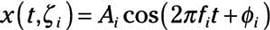where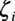corresponds to the drawing of a particular set of values from a set of possible outcomes. Relax; incorporating random parameters in your signal models is a topic left to more advanced courses.

To visualize the concepts in this section, including randomness, you can use the IPython environment with PyLab to create a plot of deterministic and random waveform examples:

```In [<b>234</b>]: t = linspace(0,5,200)
In [<b>235</b>]: x1 = 1.5*cos(2*pi*1*t + pi/3)
In [<b>237</b>]: plot(t,x1)
In [<b>242</b>]: for k in range(0,5): # loop with k=0,1,…,4
...: x2 = (1.5+rand(1)-0.5))*cos(2*pi*1*t + pi/2*rand(1)) # rand()= is uniform on (0,1)
...: plot(t,x2,'b')
...:```

The results are shown here, which uses a 2xPyLab subplot to stack plots.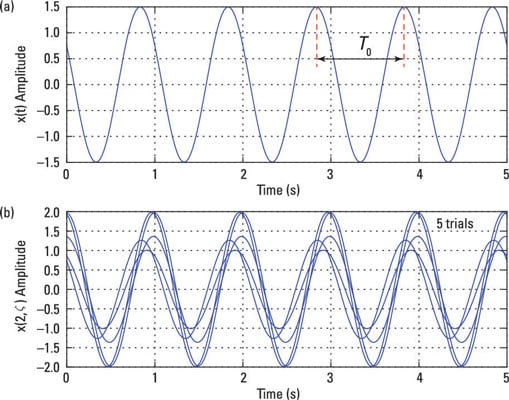Generate the deterministic sinusoid by creating a vector of time samples, t, running from zero to five seconds. To create the signal, x1 in this case, these values were chosen for the waveform parameters: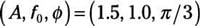For the random signal case, A is nominally 1.5, but a random number uniform over (–0.5, 0.5) is added to A, making the composite sinusoid amplitude random. The frequency is fixed at 1.0, and the phase is uniform over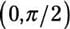Five realizations of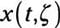are created using a for loop.